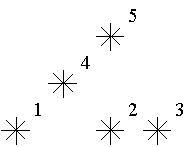# poj2352:Stars(单点更新，树状数组做法)

Stars
 Time Limit: 1000MS Memory Limit: 65536K Total Submissions: 40264 Accepted: 17491

Description

Astronomers often examine star maps where stars are represented by points on a plane and each star has Cartesian coordinates. Let the level of a star be an amount of the stars that are not higher and not to the right of the given star. Astronomers want to know the distribution of the levels of the stars.For example, look at the map shown on the figure above. Level of the star number 5 is equal to 3 (it's formed by three stars with a numbers 1, 2 and 4). And the levels of the stars numbered by 2 and 4 are 1. At this map there are only one star of the level 0, two stars of the level 1, one star of the level 2, and one star of the level 3.You are to write a program that will count the amounts of the stars of each level on a given map.

Input

The first line of the input file contains a number of stars N (1<=N<=15000). The following N lines describe coordinates of stars (two integers X and Y per line separated by a space, 0<=X,Y<=32000). There can be only one star at one point of the plane. Stars are listed in ascending order of Y coordinate. Stars with equal Y coordinates are listed in ascending order of X coordinate.

Output

The output should contain N lines, one number per line. The first line contains amount of stars of the level 0, the second does amount of stars of the level 1 and so on, the last line contains amount of stars of the level N-1.

Sample Input

```5
1 1
5 1
7 1
3 3
5 5```

Sample Output

```1
2
1
1
0```

Hint

This problem has huge input data,use scanf() instead of cin to read data to avoid time limit exceed.

Source

AC之。

```/*
Source Code

Problem: 2352		User: aclolicon
Memory: 660K		Time: 375MS
Language: G++		Result: Accepted
Source Code
*/
#include
#include
#define MAXN 35000
int l[MAXN], d[MAXN];
int n, x, y;
int lowbit(int x) {
return x & (-x);
}
void update(int i){
while(i <= MAXN){
d[i]++;
i += lowbit(i);
}
}

int sum(int i){
int s = 0;
while(i > 0){
s += d[i];
i -= lowbit(i);
}
return s;
}
int main(){
scanf("%d",&n);
memset(l, 0, sizeof(l));
memset(d, 0, sizeof(d));
for (int i = 0; i < n; i++){
scanf("%d%d", &x, &y);
x++;//处理一下
l[sum(x)]++; //因为是按顺序输入的在这里直接更新即可
update(x);//为什么要在后面update?因为防止把自己也算在内
}
for (int i = 0; i < n; i++){
printf("%dn", l[i]);
}
}```